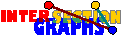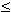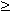# Linear k-uniform hypergraphs

A hypergraph is linear if every two hyperedges share at most one point (Note that every linear hypergraph without hyperedges of cardinality smaller than 2 is simple). Again a Krausz-type characterization is possible:A graph is the intersection graph of some (simple) linear k-uniform hypergraph if and only if there are complete subgraphs such that every vertex lies in at most k of them (and no two in the same k of them) and every edge lies in exactly one of these subgraphs.

Again the recognition problem is difficult: Recognizing intersection graphs of 3-uniform linear hypergraphs is NP-complete.

K5-e and the complement of 4K2 are intersection graphs of linear 3-uniform hypergraphs, but K6-e and the complement of 5K2 are not.

The following cruical lemma (compare the analogue for line graphs), follows by the classification of cliques in intersection graphs of 3-uniform hypergraphs. However, there is also a simpler proof:[NRSS82], [JKL97], [HK97], [MT97], Every clique with more than k2-k+1 vertices in the intersection graph G of a k-uniform linear simple hypergraph H is a star graph.
 Proof: Assume the hyperedges X1, X2,..., Xn with n > k(k-1)+1 in a linear hypergraph are pairwise intersecting. By the pigeon-hole principle, some point a of X1 is covered by at least k of the sets X2,...,Xn, say aX2,..., aXk+1. Every Xi, k+1 < in, not containg a has to intersect X1, X2,...,Xk+1 in different points, this is impossible since |Xi|= k. Thus all Xi contain a.

Therefore, other than in the general case, in the linear case all large enough cliques of the intersection graph must be star cliques. Then we can achieve polynomiality for the recognition problem by restricting the possible models:For every k3, let H be the class of k-uniform linear hypergraphs where every point lies in at least k2-k+2 hyperedges. Then intersection graphs of members of H can be recognized in polynomial time.

However, it is always more desirable to get a characterization under additional assumptions on the graph, not on the model. This is possible for k=3: In [NRSS82] it has been shown that it is possible to test graphs of minimum degree at least 69 whether or not they are intersection graphs of linear 3-uniform hypergraphs. The bound has recently independently been lowered to 19 by a different approach by two groups [JKL97] and [MT97]. Even a bound of 14 is possible:[MPST*]: There is some polynomial-time algorithm that tests graphs of minimum degree at least 14 whether or not they are intersection graphs of linear 3-uniform hypergraphs.
 Sketch of the proof for minimum degree 19: [JKL97] [MT97] So assume G is the intersection graph of some linear 3-uniform hypergraph, and assume(G)19. Key of the algorithm is the fact that, if we reveal all cliques with more than 7 vertices as star graphs, every vertex must lie in at least one of these already revealed star graphs (since its at most 19 neighbors should be covered by three star graphs). Before we list all cliques, we should however check whether G does not contain the complement of 5K2 as induced subgraph, as it should. Every vertex lying in one or two of these already revealed star cliques gets 2 respectively 1 as a label. Next we delete all edges in the the revealed star cliques. Every vertex that lied in three of them should be isolated now, and is also deleted. For the resulting vertex-labeled graph G´ we check whether it is the intersection graph of some linear hypergraph where every Sx should have as many elements (1 or 2) as the label of x indicates. This is essentially recognition of line graphs, compare [JKL97] and [MT97], and can be done in linear time.

Note that this approach is not extendable to higher k.

Back to the start page for intersection graphs.

Erich Prisner